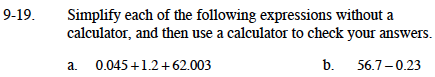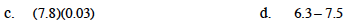### Home > MC1 > Chapter 9 > Lesson 9.1.2 > Problem9-19

9-19.Make sure you add the parts that are like.
You can think of 0.045 as

$\frac{4}{100}\text{ and } \frac{5}{1000}\text{ or is }\frac{45}{1000}.$

How can you combine this with the other numbers?
Be sure to show your work.

$\text{You can think of 56.7 as } 56\frac{7}{10}\text{ and 0.23 as } \frac{23}{100}.$

How can you subtract 23-hundredths from 70-hundredths?0.234

Since 7.5 is greater than 6.3, your answer should be less than zero.

−1.2